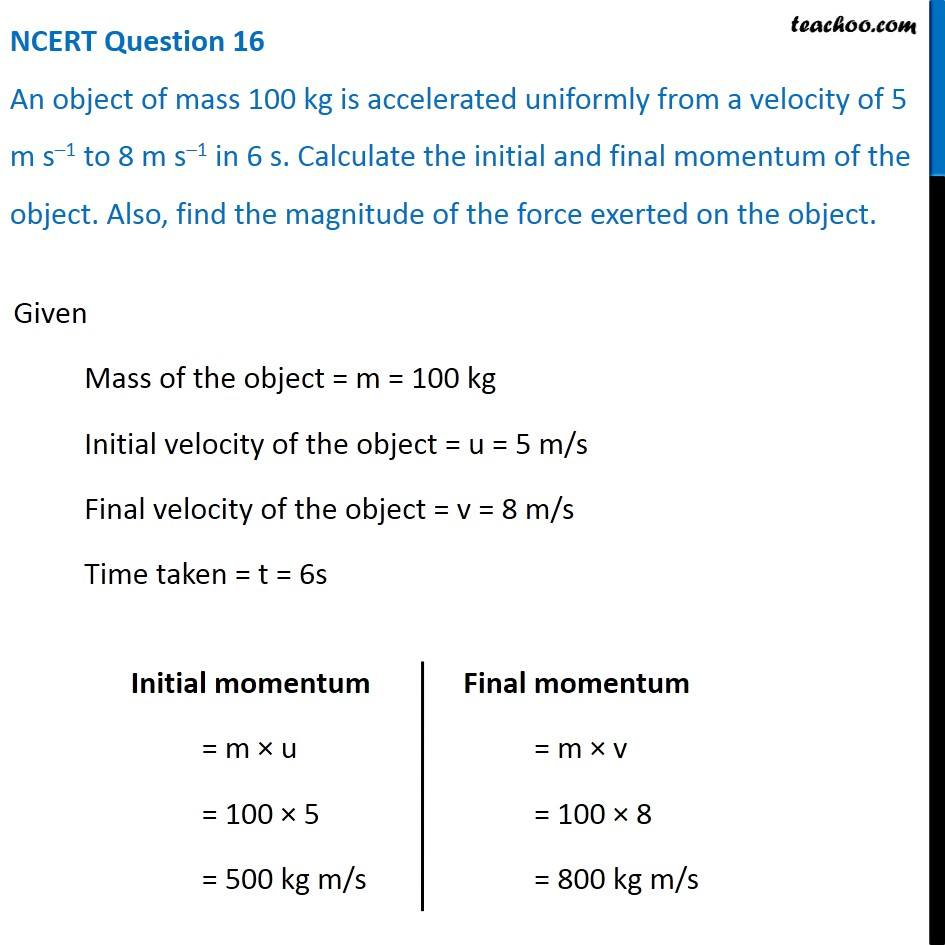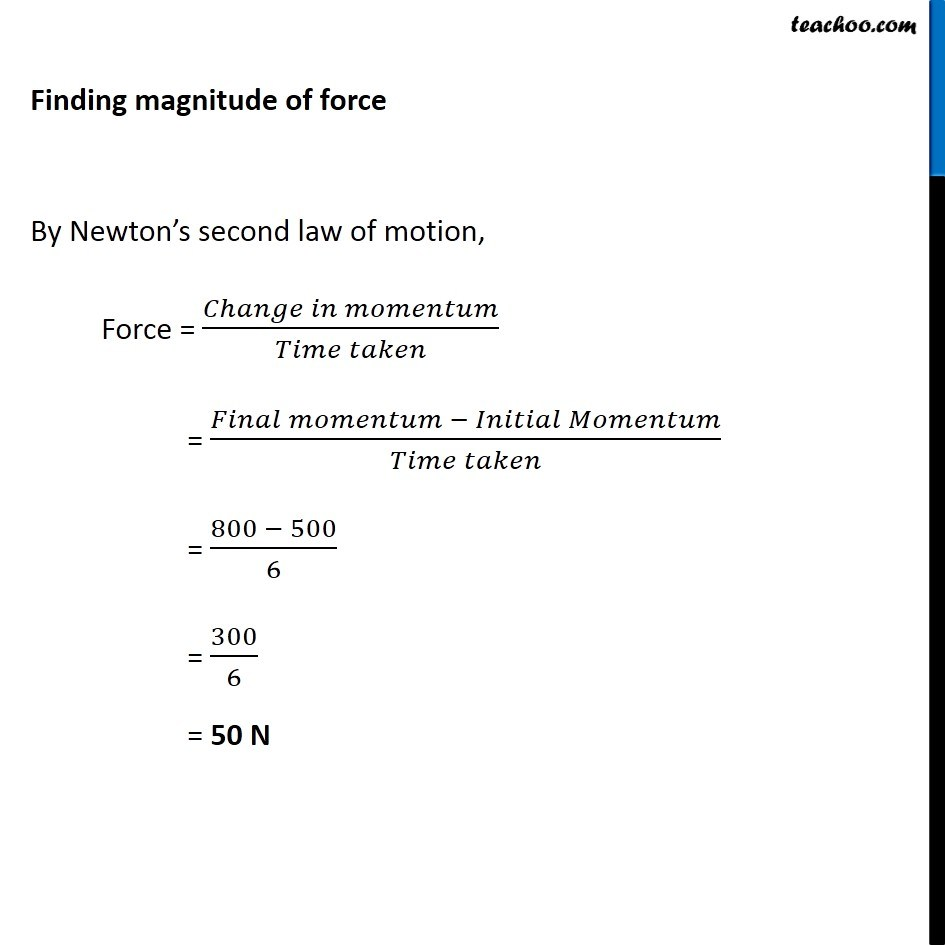NCERT Questions

Class 9
Chapter 9 Class 9 - Force and Laws Of MotionGet live Maths 1-on-1 Classs - Class 6 to 12

### Transcript

NCERT Question 16 An object of mass 100 kg is accelerated uniformly from a velocity of 5 m s–1 to 8 m s–1 in 6 s. Calculate the initial and final momentum of the object. Also, find the magnitude of the force exerted on the object. Given Mass of the object = m = 100 kg Initial velocity of the object = u = 5 m/s Final velocity of the object = v = 8 m/s Time taken = t = 6s Initial momentum = m × u = 100 × 5 = 500 kg m/s Final momentum = m × v = 100 × 8 = 800 kg m/s Finding magnitude of force By Newton’s second law of motion, Force = (𝐶ℎ𝑎𝑛𝑔𝑒 𝑖𝑛 𝑚𝑜𝑚𝑒𝑛𝑡𝑢𝑚)/(𝑇𝑖𝑚𝑒 𝑡𝑎𝑘𝑒𝑛) = (𝐹𝑖𝑛𝑎𝑙 𝑚𝑜𝑚𝑒𝑛𝑡𝑢𝑚 − 𝐼𝑛𝑖𝑡𝑖𝑎𝑙 𝑀𝑜𝑚𝑒𝑛𝑡𝑢𝑚)/(𝑇𝑖𝑚𝑒 𝑡𝑎𝑘𝑒𝑛) = (800 − 500)/6 = 300/6 = 50 N